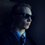# $\epsilon = \frac{1}{\infty} = 0$

Again inspired by a Numberphile video, I started to ponder the similarities between an "infinitesimal", often represented as $\epsilon$, and the number $0$.

Without diving too deeply into the meanings of infinitesimals (the video does this much better than I can), a basic definition of $\epsilon$ is this:

$0 < \epsilon < r$

Where $r$ is any and all of the real numbers. In other words, $\epsilon$ is smaller than all other numbers, yet it is greater than $0$.

Now please note that the following is more pondering and speculation than rigorous exposition. :)

What I realized is that if $\epsilon$ represents an "infinitely small" number, and if, as I recently speculated in another discussion, $\frac{1}{0}$ is really equal to $\infty$, than perhaps we should just equate $\epsilon$ to $0$.

As an equation, this could be stated as:

$\epsilon = \frac{1}{\infty} = 0$.

Another thing to note is that I am not proposing that $\epsilon$, as it has been defined by mathematicians, is equal to $0$. This is more of a reinterpretation of what an infinitesimal is. In this way, I have really just redefined an infinitesimal as actually representing $0$. Just as infinity represents infinitely much, or everything, perhaps an infinitesimal represents infinitely little, or nothing.

This equation results in several satisfying results, but the result which most interested me was this: One of the axioms of infinitesimals is that there can be multiples of $\epsilon$, so $2\epsilon$, $3\epsilon$, and so on. The $\epsilon = \frac{1}{\infty} = 0$ parallel to this would be that there can be multiples of $\frac{1}{\infty}$, so $\frac{2}{\infty}$, $\frac{3}{\infty}$, and so on.

Does anyone have any ideas/opinions on this? If so, I would love to hear them!Note by David Stiff
1 year, 8 months ago

This discussion board is a place to discuss our Daily Challenges and the math and science related to those challenges. Explanations are more than just a solution — they should explain the steps and thinking strategies that you used to obtain the solution. Comments should further the discussion of math and science.

When posting on Brilliant:

• Use the emojis to react to an explanation, whether you're congratulating a job well done , or just really confused .
• Ask specific questions about the challenge or the steps in somebody's explanation. Well-posed questions can add a lot to the discussion, but posting "I don't understand!" doesn't help anyone.
• Try to contribute something new to the discussion, whether it is an extension, generalization or other idea related to the challenge.

MarkdownAppears as
*italics* or _italics_ italics
**bold** or __bold__ bold
- bulleted- list
• bulleted
• list
1. numbered2. list
1. numbered
2. list
Note: you must add a full line of space before and after lists for them to show up correctly
paragraph 1paragraph 2

paragraph 1

paragraph 2

[example link](https://brilliant.org)example link
> This is a quote
This is a quote
    # I indented these lines
# 4 spaces, and now they show
# up as a code block.

print "hello world"
# I indented these lines
# 4 spaces, and now they show
# up as a code block.

print "hello world"
MathAppears as
Remember to wrap math in $$ ... $$ or $ ... $ to ensure proper formatting.
2 \times 3 $2 \times 3$
2^{34} $2^{34}$
a_{i-1} $a_{i-1}$
\frac{2}{3} $\frac{2}{3}$
\sqrt{2} $\sqrt{2}$
\sum_{i=1}^3 $\sum_{i=1}^3$
\sin \theta $\sin \theta$
\boxed{123} $\boxed{123}$